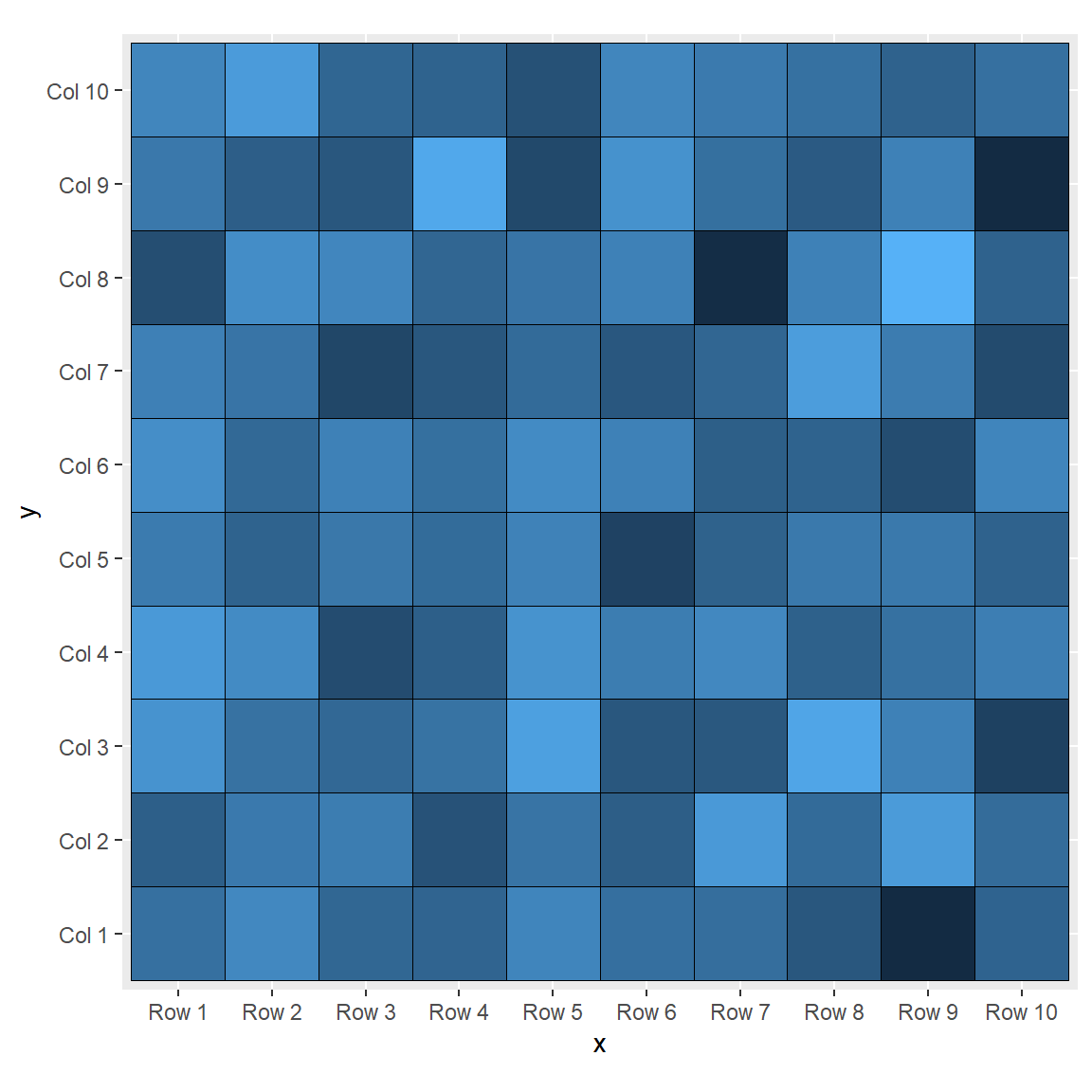# Heat map in ggplot2

## Sample data

Given a numerical matrix you will need to transform it into a data frame that ggplot2 can understand. For that purpose you can use the `melt` function from `reshape` package.

``````# install.packages("reshape")
library(reshape)

# Data
set.seed(8)
m <- matrix(round(rnorm(200), 2), 10, 10)
colnames(m) <- paste("Col", 1:10)
rownames(m) <- paste("Row", 1:10)

# Transform the matrix in long format
df <- melt(m)
colnames(df) <- c("x", "y", "value")``````

## Heat map with `geom_tile`

A heap map in ggplot2 can be created with `geom_tile`, passing the categorical variables to `x` and `y` arguments and the continuous variable to `fill` argument of `aes`.

``````# install.packages("ggplot2")
library(ggplot2)

ggplot(df, aes(x = x, y = y, fill = value)) +
geom_tile()``````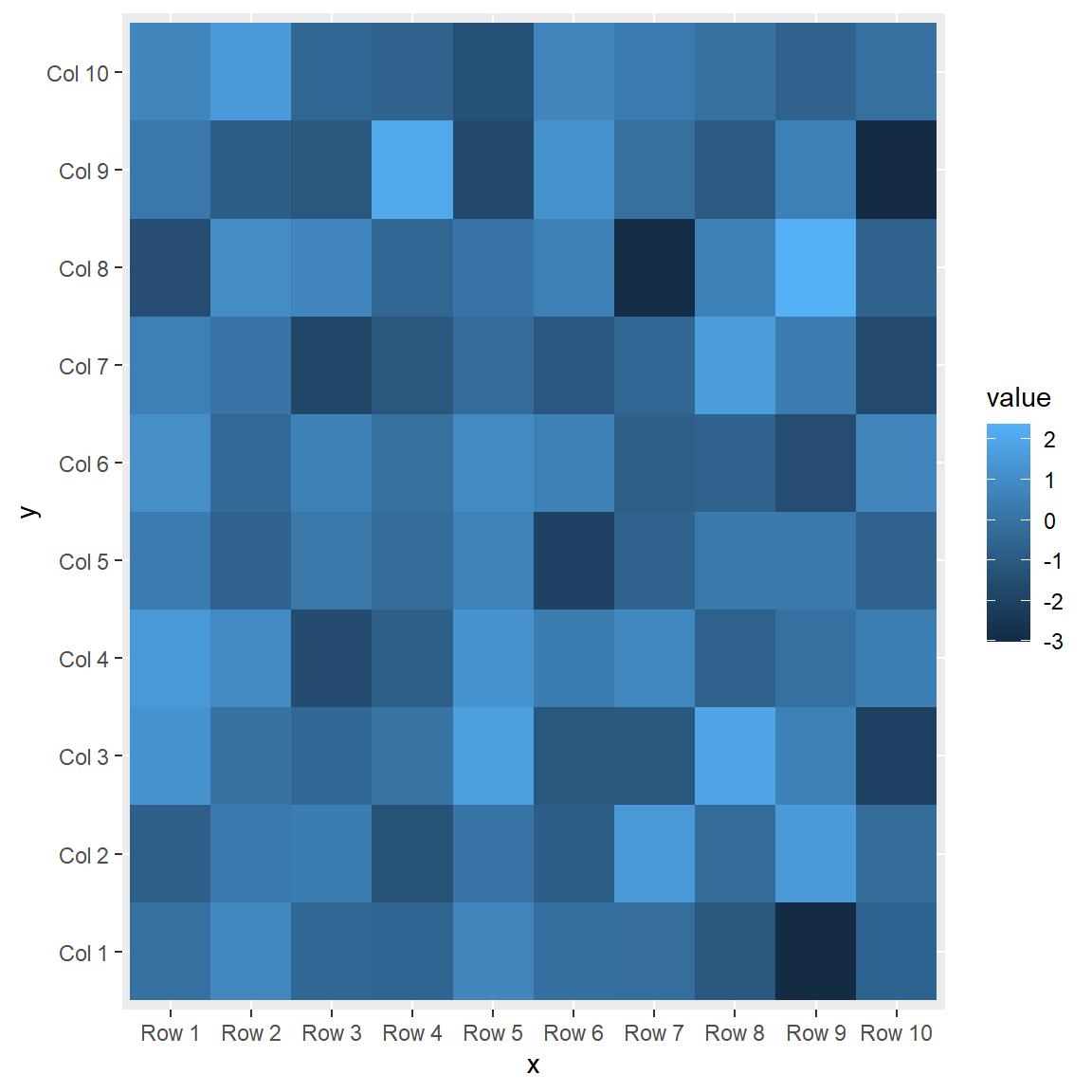Square tiles

Note that depending on the plotting windows size the tiles might not be squared. If you want to keep them squared use `cood_fixed`.

``````# install.packages("ggplot2")
library(ggplot2)

ggplot(df, aes(x = x, y = y, fill = value)) +
geom_tile() +
coord_fixed()``````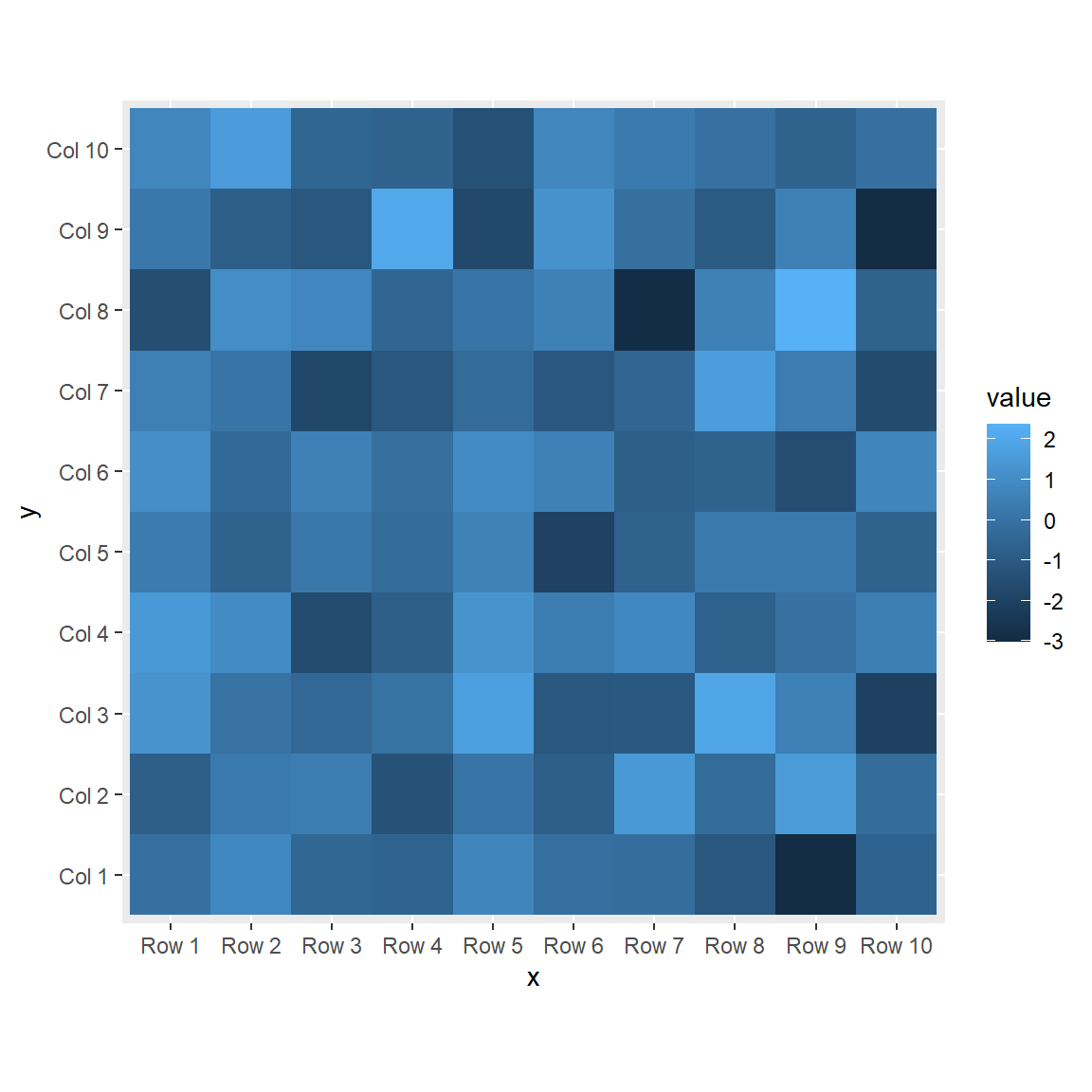Border customization

You can customize the border color, line width and line style of the tiles with `color`, `lwd` and `linetype`, respectively.

``````# install.packages("ggplot2")
library(ggplot2)

ggplot(df, aes(x = x, y = y, fill = value)) +
geom_tile(color = "white",
lwd = 1.5,
linetype = 1) +
coord_fixed()``````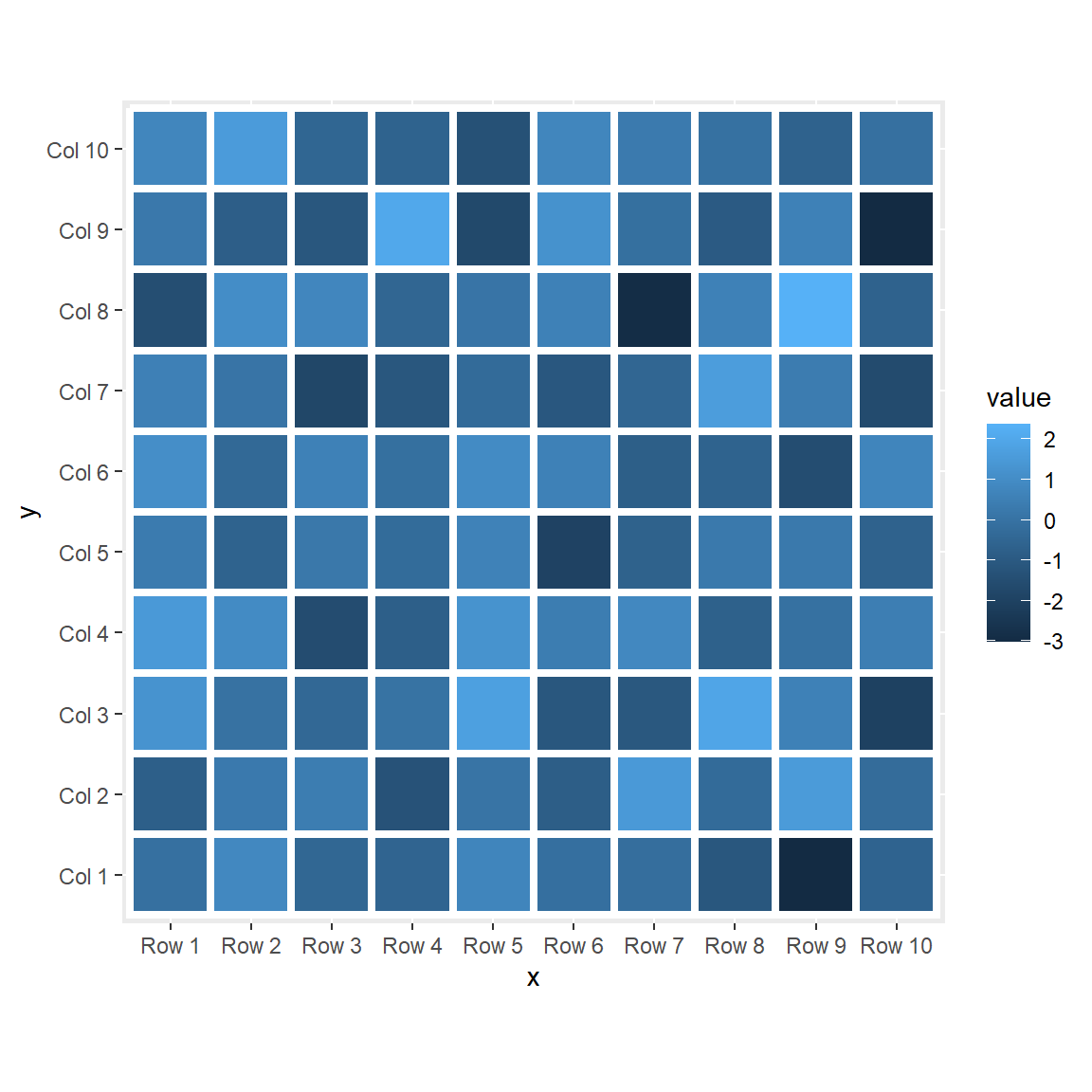In addition, you can add the values over the tiles with `geom_text`, passing the numerical variable to the `label` argument of the `aes` function.

``````# install.packages("ggplot2")
library(ggplot2)

ggplot(df, aes(x = x, y = y, fill = value)) +
geom_tile(color = "black") +
geom_text(aes(label = value), color = "white", size = 4) +
coord_fixed()``````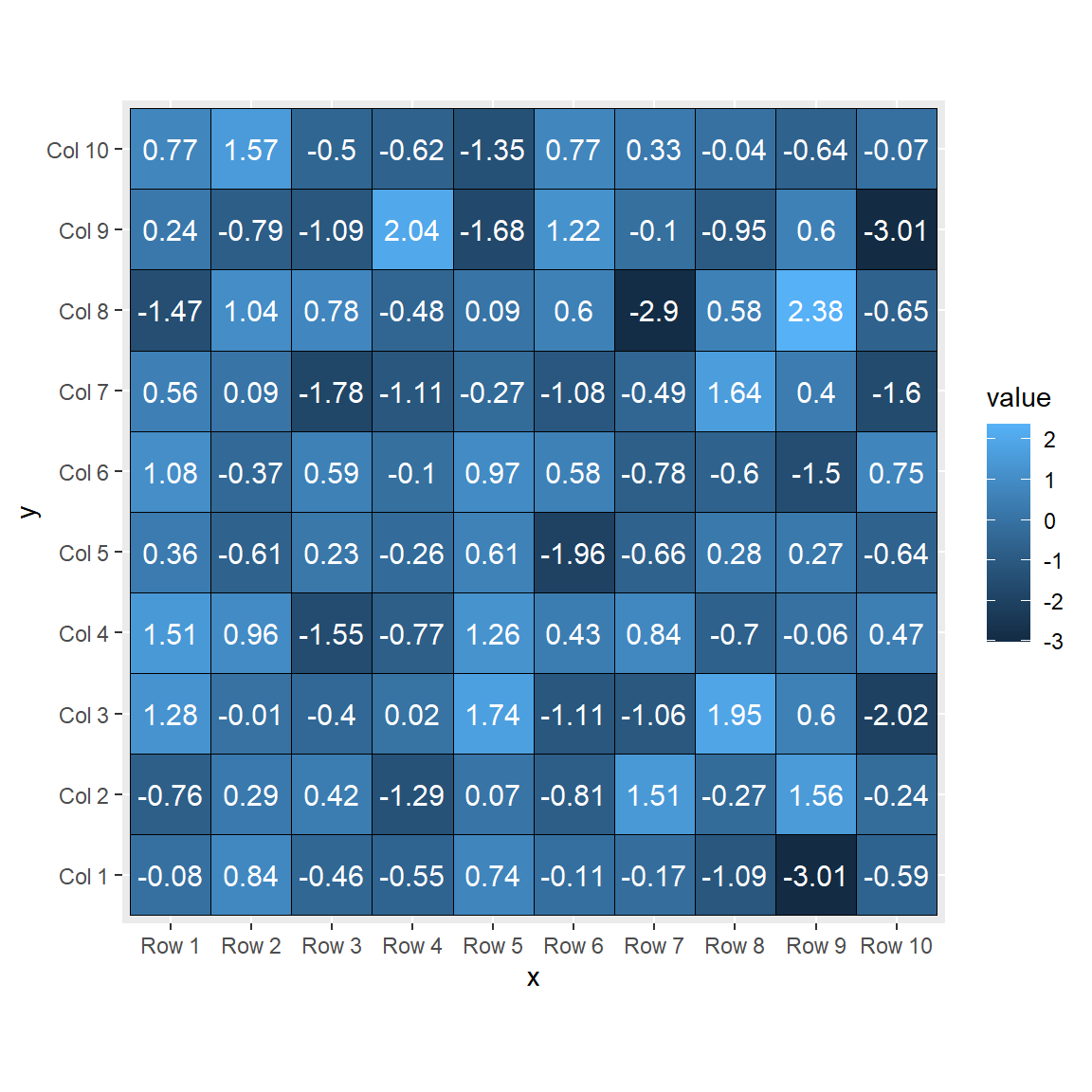## Color palette

There three ways to change the default color palette used when creating the heat map: using `scale_fill_gradient`, `scale_fill_gradient2` or `scale_fill_gradientn`.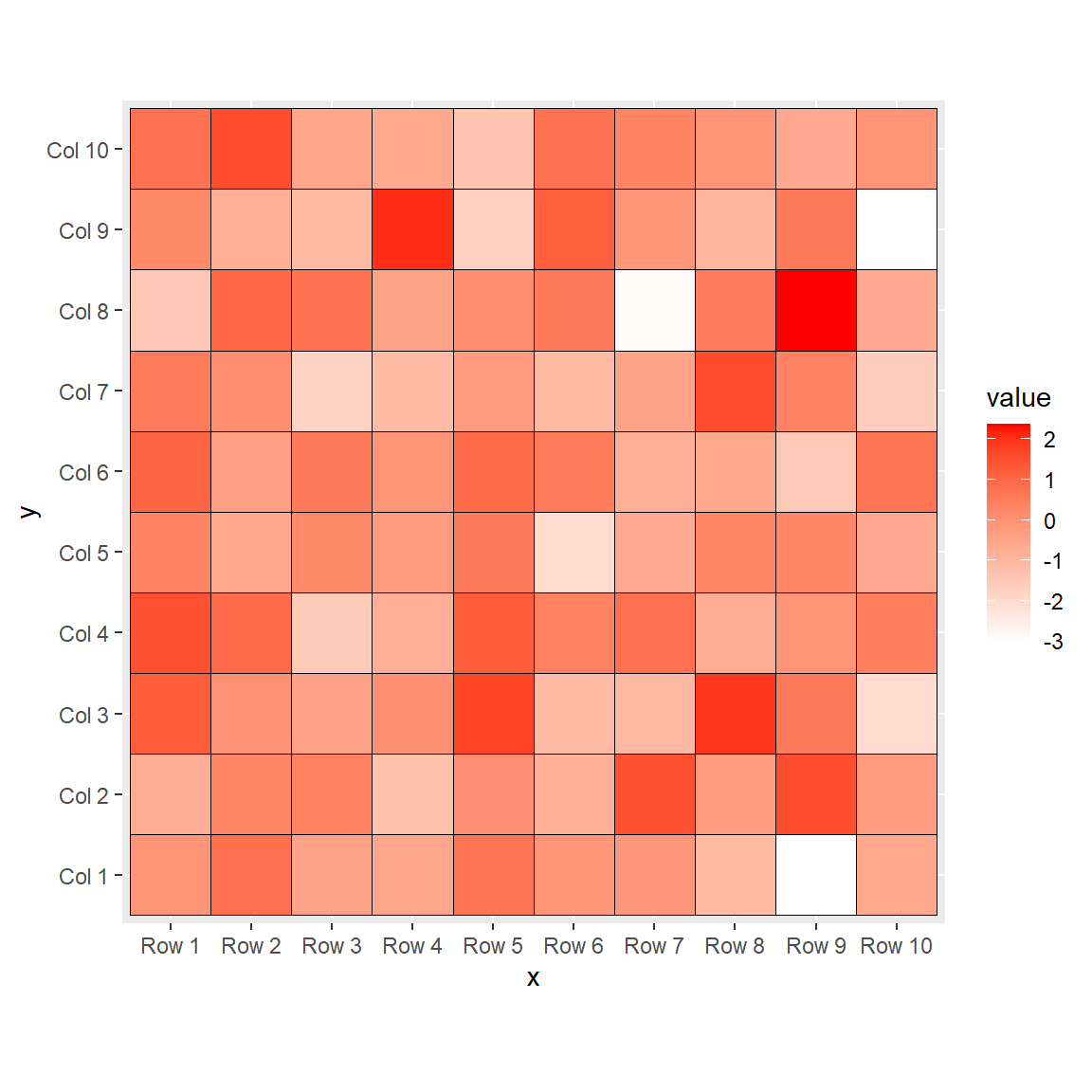This function allows changing the colors, setting a lower and a higher color to represent the values of the heat map.

``````# install.packages("ggplot2")
library(ggplot2)

ggplot(df, aes(x = x, y = y, fill = value)) +
geom_tile(color = "black") +
scale_fill_gradient(low = "white", high = "red") +
coord_fixed()``````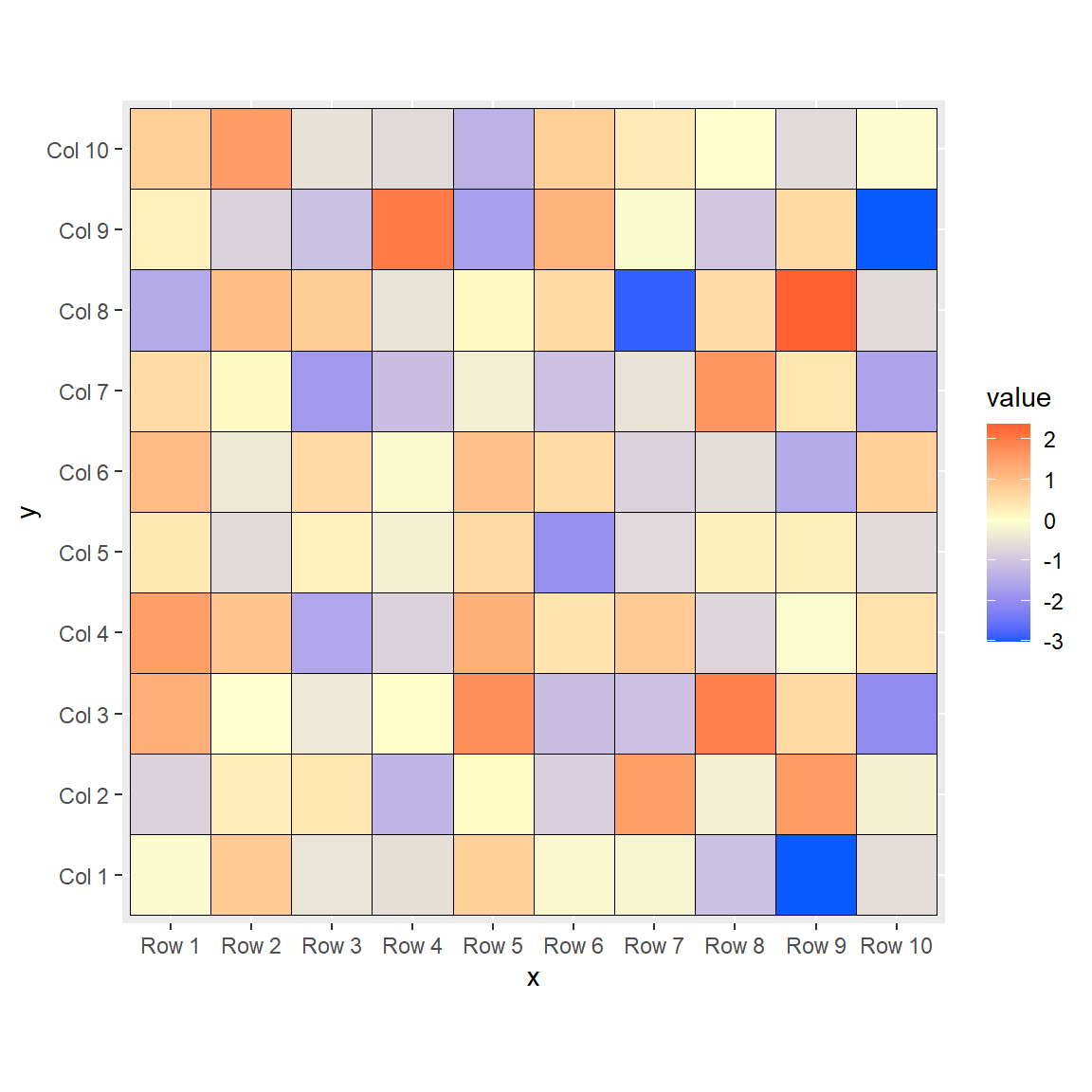If you want to add a mid color you can use `scale_fill_gradient2`, which includes the `mid` argument.

``````# install.packages("ggplot2")
library(ggplot2)

ggplot(df, aes(x = x, y = y, fill = value)) +
geom_tile(color = "black") +
mid = "#FFFFCC",
high = "#FF0000") +
coord_fixed()``````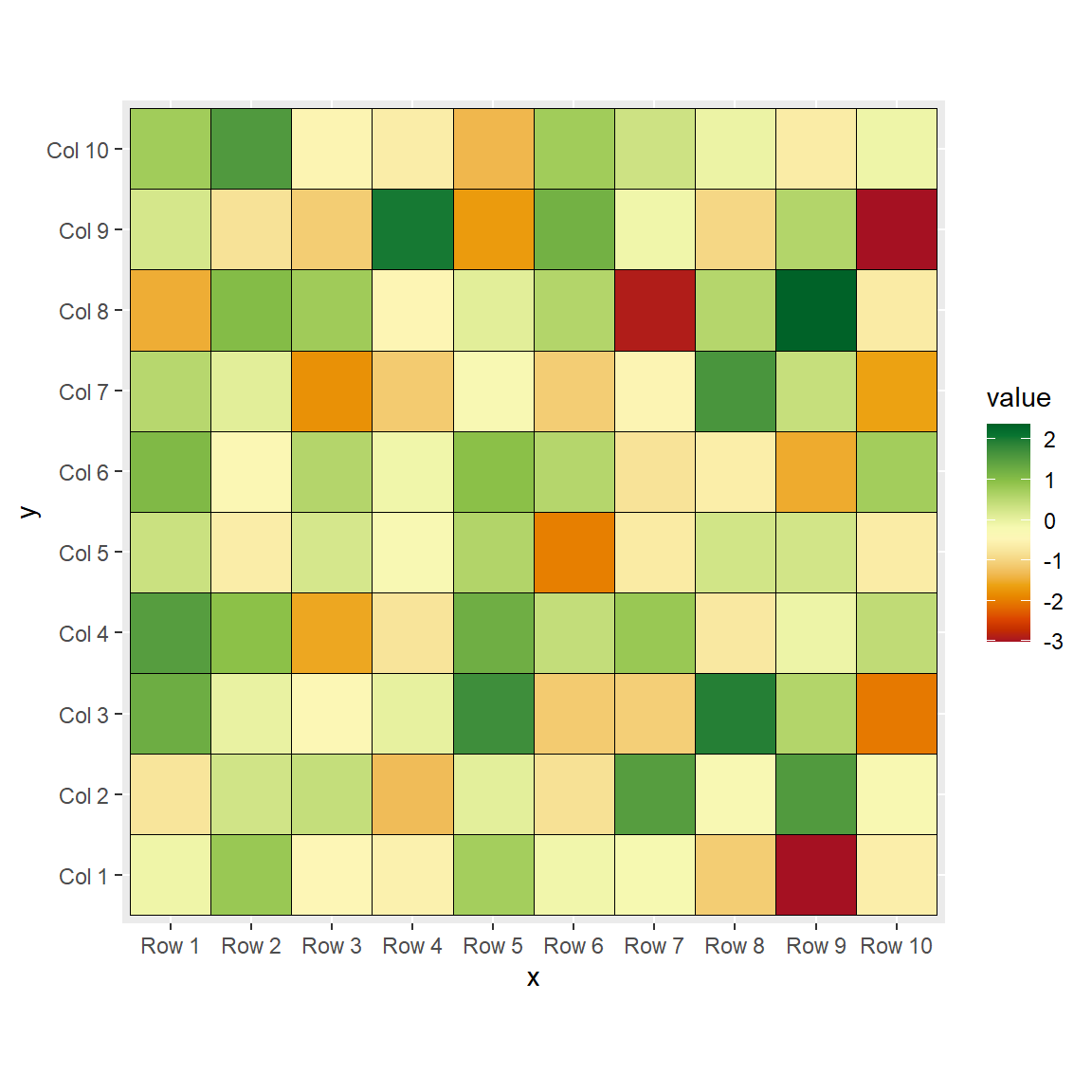Finally, you can also use a custom color palette with `scale_fill_gradientn`, which allows passing n colors to the `colors` argument. In this example we are passing 20 colors of the `"RdYlGn"` palette.

``````# install.packages("ggplot2")
library(ggplot2)

ggplot(df, aes(x = x, y = y, fill = value)) +
geom_tile(color = "black") +
scale_fill_gradientn(colors = hcl.colors(20, "RdYlGn")) +
coord_fixed()``````

## Legend customization

Width and height

You can change the width and the height of the legend color bar with the following code:

``````# install.packages("ggplot2")
library(ggplot2)

ggplot(df, aes(x = x, y = y, fill = value)) +
geom_tile(color = "black") +
coord_fixed() +
guides(fill = guide_colourbar(barwidth = 0.5,
barheight = 20))``````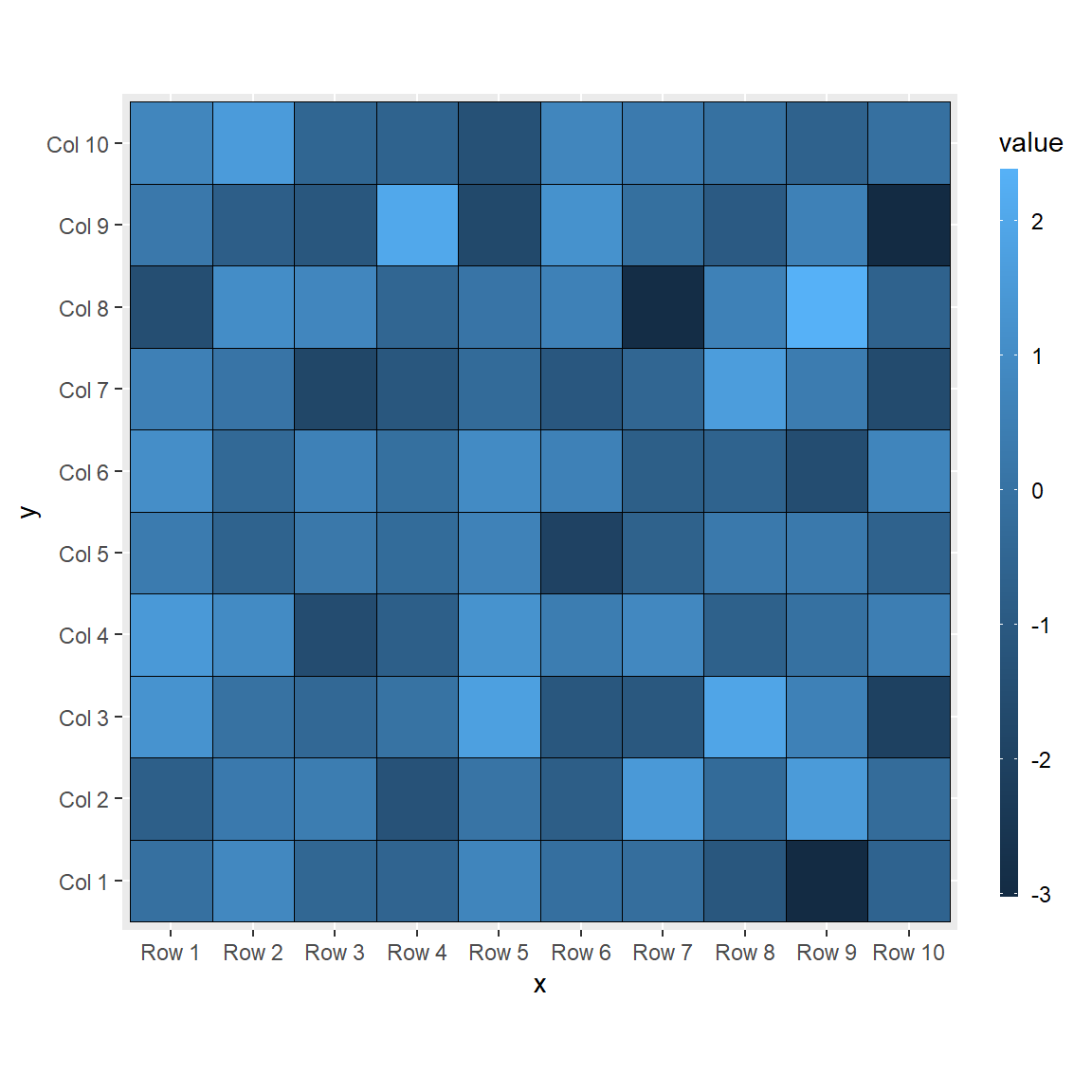Change the title

The default title of the legend is the name of the continuous variable of the data frame. If you want to change it pass a string to the `title` argument of the `guide_colourbar` function.

``````# install.packages("ggplot2")
library(ggplot2)

ggplot(df, aes(x = x, y = y, fill = value)) +
geom_tile(color = "black") +
coord_fixed() +
guides(fill = guide_colourbar(title = "Title"))``````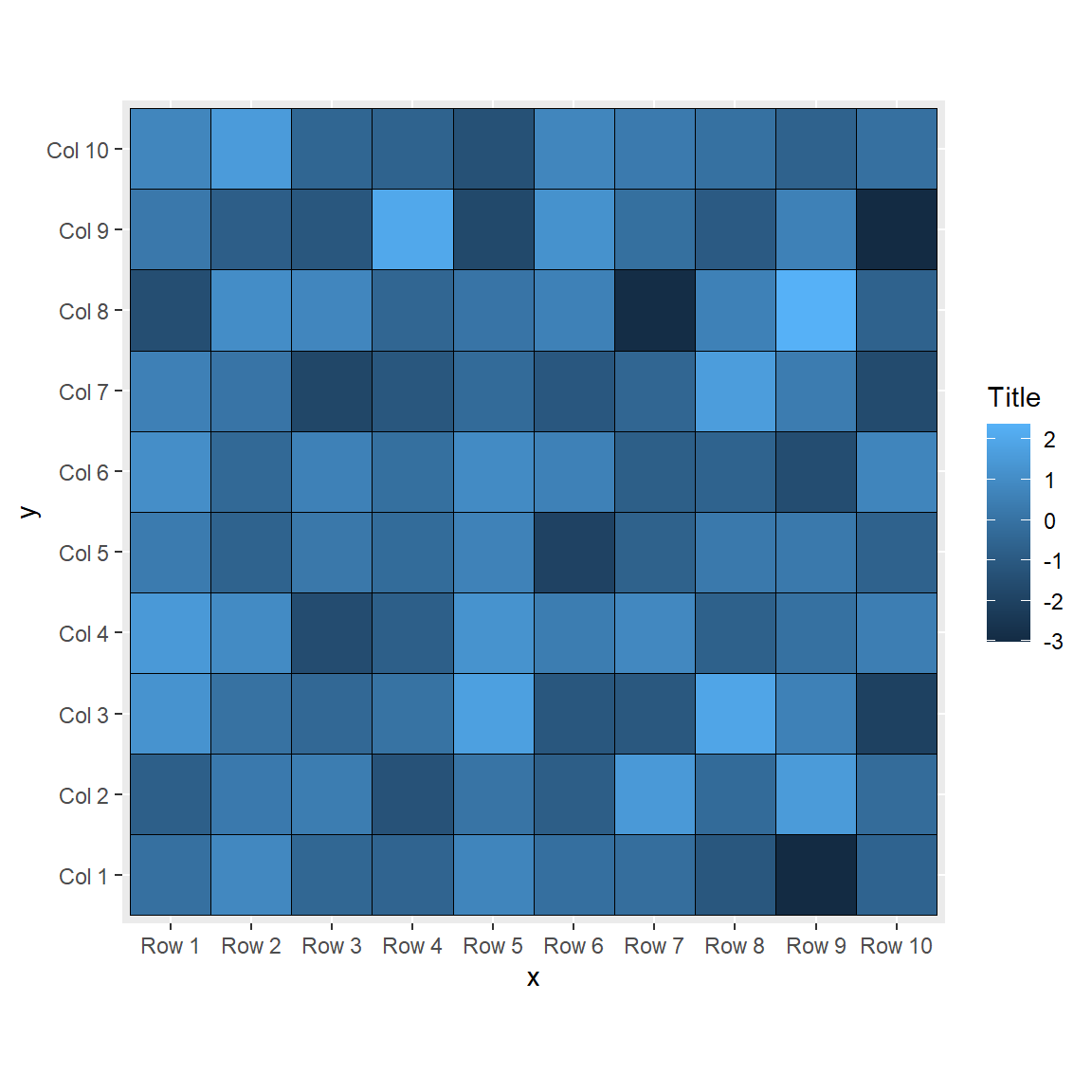Remove the labels and the ticks

You can also remove the labels and/or the ticks of the legend, setting the corresponding arguments to `FALSE`.

``````# install.packages("ggplot2")
library(ggplot2)

ggplot(df, aes(x = x, y = y, fill = value)) +
geom_tile(color = "black") +
coord_fixed() +
guides(fill = guide_colourbar(label = FALSE,
ticks = FALSE))``````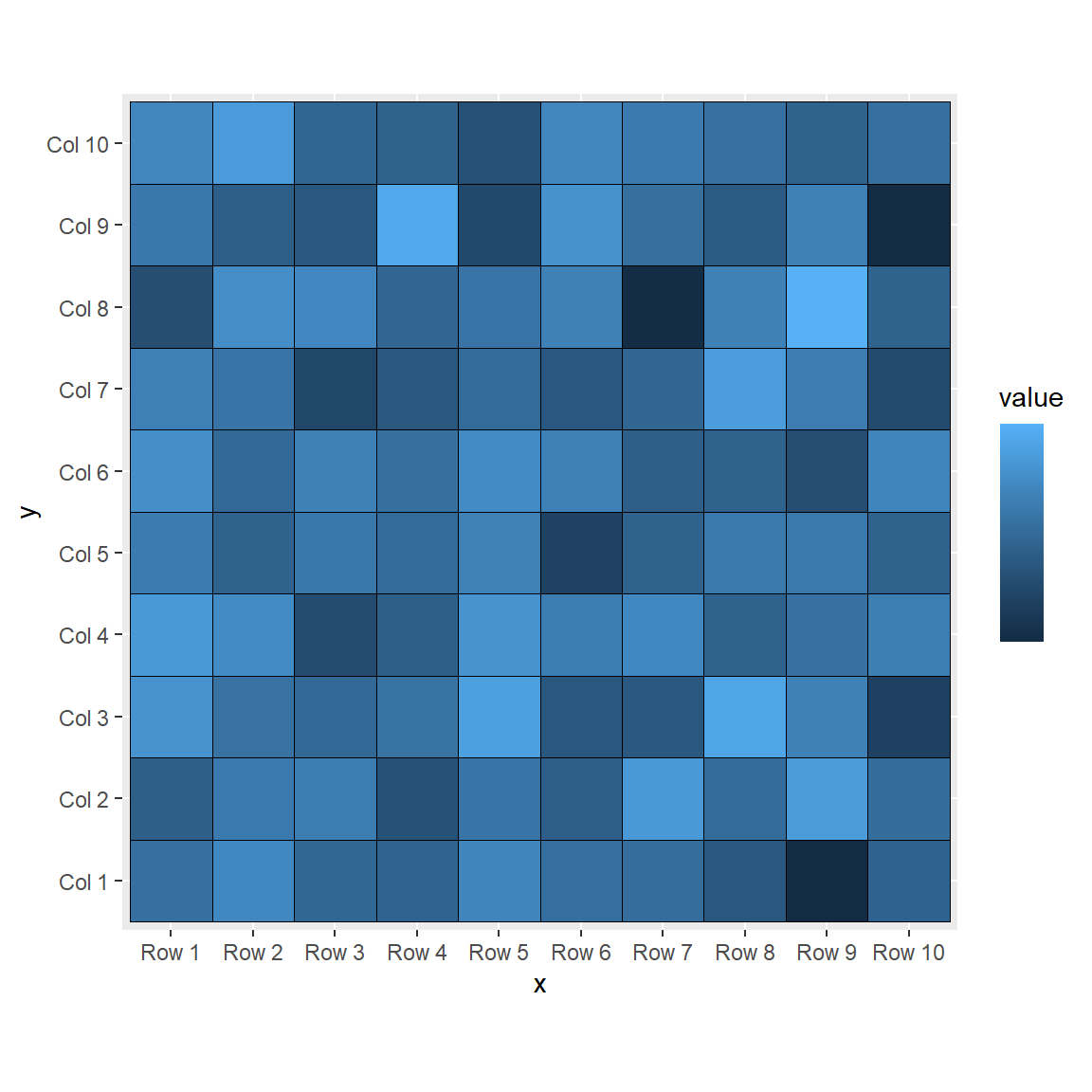Remove the legend

Finally, it you want to get rid of the legend you can set its position to `"none"`.

``````# install.packages("ggplot2")
library(ggplot2)

ggplot(df, aes(x = x, y = y, fill = value)) +
geom_tile(color = "black") +
coord_fixed() +
theme(legend.position = "none")``````